# SAT Math Multiple Choice Question 279: Answer and Explanation

### Test Information

Question: 279

9. Which of the following functions has a domain of x ≥ 2?

• A. f(x) = -x2 + 2
• B.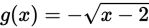• C.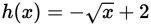• D.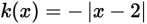Getting to the Answer: The domain in the question is restricted to numbers greater than or equal to 2, so you can immediately eliminate A and D-the domain of a quadratic function and an absolute function is all real numbers. To choose between (B) and C, you can draw a quick sketch or think about how transformations affect the domain of each function. The domain of the parent function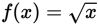is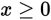(nonnegative numbers). In (B), the parent function is reflected vertically across the horizontal axis (which doesn't change the domain) and then shifted to the right 2 (making the domain x ≥ 2), so (B) is correct. Note that in C, the function is reflected across the horizontal axis and then shifted up 2 units, which adds 2 to the range of the function, not the domain.